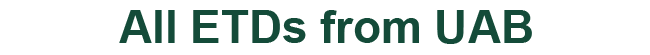### Multi-Coefficient Dirichlet Neumann Type Elliptic Inverse Problems With Application to Reflection Seismology

#### Authors

Ian Knowles

Thomas Jannett

Tsun-Zee Mai

S S Ravindran

Gunter Stolz

Gilbert Weinstein

Dissertation

2009

#### Degree Name by School

Doctor of Philosophy (PhD) College of Arts and Sciences

#### Abstract

lliptic inverse problems requiring multi-coefficient recovery from boundary measurements are encountered in several areas of science and engineering. We present a fully non-linear variational approach to the aforementioned problem. Our investigation is motivated by the reflection seismology problem of recovering the subsurface parameters from acoustic measurements on the surface. By incorporating measurements of the normal derivative of pressure at the surface, and through a finite Laplace transform, the inverse recovery of parameters ρ, κ and γ from the hyperbolic acoustic equation 1 ρ(x)κ2(x) (ptt + γ(x)pt) − ∇ · 1 ρ(x) ∇p = F(x, t) on

p(x, t) = ζ(x, t) on �� ∂p ∂n = χ(x, t) on �� is reformulated as the recovery of parameters A, B, C, R and S from the elliptic equation −∇ · (A(x)∇u) + (λ2(x)B(x) + λC(x))u = −e−λ(λR(x) + S(x)) + ˆ F(x, λ) with a known Dirichlet and partially known Neumann data. The coefficients A,B,C,R & S of the transformed elliptic equation are then recovered as the (conjectured) unique stationary points of an appropriate functional by using Neuberger gradient descent. As a special case, we apply our algorithm to the electrical impedance tomography problem of recovering (discontinuous) electrical conductivity. The use of Neumann Neuberger gradient allow us to weaken the conductivity assumption on the boundary. ii For the seismic recovery problem our approach yields a full waveform technique for inversion and our descent algorithm facilitates stable recovery of multiple coefficients from boundary measurements.

COinS